### Home > MC1 > Chapter 6 > Lesson 6.3.3 > Problem6-147

6-147.
1. . Without a calculator, find the following products. Homework Help ✎

1.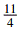of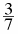2.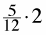3. 4.16(0.2)

4.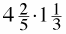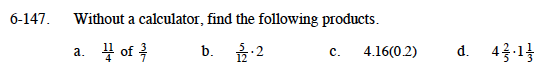Refer to the Math Notes box to the right if you are having trouble.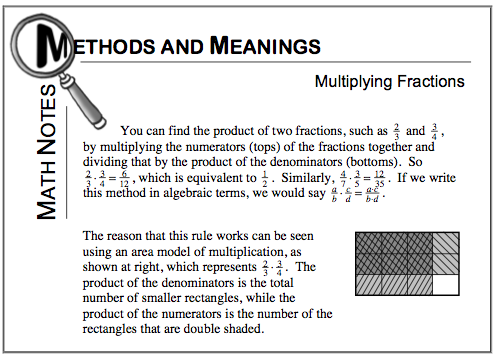Treat 2 as the fraction

$\frac{2}{1}.$

Try representing these portions as fractions.

0.832

$\frac{88}{15}=5\frac{13}{15}$

Begin by converting each mixed number to a fraction greater than one and then multiply.